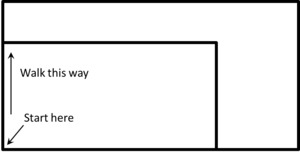# How Big is a Foot?

Alignments to Content Standards: 2.MD.A

#### Materials

• How Big is a Foot? by Rolf Myller• Enough 12-inch (one foot) rulers for every student or pair of students

• One yardstick

• Twelve one-inch strips of paper

• Three one-foot strips of paper

• Worksheets (attached)

#### Actions

• The teacher reads How Big is a Foot? to the class, stopping at the following key moments to ask the students questions.
• At one point the characters are asking, "How big is a bed?" Ask the students how they would describe the size of a bed.
• At another point the question arises, "Why was the bed too small for the queen?" Ask the students why they think the bed was too small for the queen.
• At the end the bed fits the queen perfectly. Ask the students, "Why did the bed fit perfectly?"

These discussion points are meant to encourage the students to develop their own understanding of what a foot is and why having a standard unit of measurement is necessary.

• (Optional) The class can re-enact what happened in the story. First, the teacher paces off a rectangle that is three "teacher feet" wide and 6 "teacher feet" long by stepping heel-to-toe. The teacher should mark the floor with a piece of masking tape at the corners of the rectangle along the way, and then mark each side length of the rectangle with a long strip of masking tape. Then ask a students to do the same, starting from the same corner and heading in the same direction as the teacher. The rectangles should look something like this:• After finishing the story, the teacher should show the class the one-inch strips of paper, the one-foot strips of paper, the ruler that shows one foot, and the yard stick. Lay the 12 one-inch strips of paper end-to-end and show they are the same length as a one-foot strip of paper. Measure them with the one-foot ruler. Then lay the three one-foot strips of paper end-to-end and measure them with the yard stick. Then point out that every length called a foot has 12 inches in it, and that a foot can be laid end-to-end to see how many feet long something is, just as the apprentice did at the end of the book.
• The students complete their worksheets by finding items in the classroom about a foot long and about two feet long. Then they approximate how large a bed would need to be for a partner. Students can work with partners throughout the scavenger hunt if desired.
• After students finish measuring, they should discuss as a whole group how they measured the various objects in the classroom and how they determined the dimensions of their partner's bed. The class should talking about why we use standard units of measure.

## IM Commentary

The purpose of this task is to understand the idea of a standard unit of measure and why we use them. It also reinforces the idea that we measure lengths by placing units end-to-end.

Depending on the size of items in the classroom that students are measuring, the teacher may need to point out that students should find items to measure that are ABOUT one foot or ABOUT two feet instead of exactly one or two feet long.

Here is a link to a video of a student reading the book:

## Attached Resources

• How Big is a Foot? worksheet
• ## Solution

Solutions will vary.